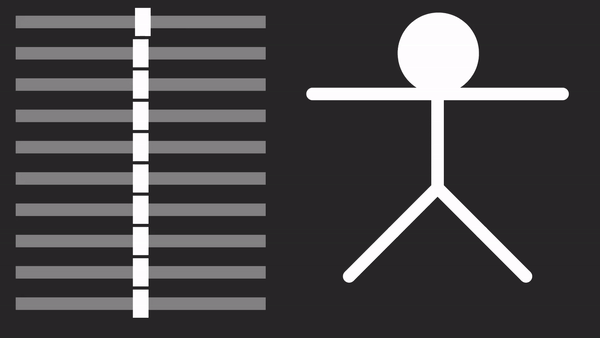# Busting Moves with DanceNet AI

Inspired by STEM-focused YouTuber carykh, Indian developer Jaison Saji has produced a deep network system, DanceNet, that can automatically generate dance moves. Synced used DanceNet to produce a short clip (below) with code published on Github.

Inspired by STEM-focused YouTuber carykh, Indian developer Jaison Saji has produced a deep network system, DanceNet, that can automatically generate dance moves. Synced used DanceNet to produce a short clip (below) with code published on Github. Interested readers can use the system to improve this work or create their own.

DanceNet uses a variational autoencoder (VAE) to automatically generate thousands of single dance pose pictures, then sequentially connect them to produce vigorous dance movements through joint training on Long Short-Term Memory (LSTM) and Mixture Density Networks (MDN).

VAE is a commonly used generative model with two parts: an encoder transfers the image into a dense representation that has few dimensions and occupies less space than the original source and stores latent information about the input; while a decoder transfers dense-represented code back to its corresponding image.

According to Saji’s code, the DanceNet encoder model consists of three convolutional layers and one fully connected layer. Source dataset images first have their backgrounds removed and then pass to the encoder to form multiple 128-dimensional informative vectors containing latent variables that follow a unit Gaussian distribution, where z_mean represents their average, and z_log_var represents their logarithm of variance.

```input_img = Input(shape=(120,208,1))
x = MaxPooling2D(pool_size=2)(x)
x = MaxPooling2D(pool_size=2)(x)
x = MaxPooling2D(pool_size=2)(x)
shape = K.int_shape(x)
x = Flatten()(x)
x = Dense(128,kernel_initializer='glorot_uniform')(x)

z_mean = Dense(latent_dim)(x)
z_log_var = Dense(latent_dim)(x)
z = Lambda(sampling, output_shape=(latent_dim,), name="z")([z_mean,z_log_var])

encoder = Model(input_img, [z_mean, z_log_var,z], name="encoder")```

The next step is to reconstruct the vectors to the original images as closely as possible. To accomplish this Saji integrates one fully connected layer and four convolutional layers with three upsampling layers in the DanceNet decoder model.

``````latent_inputs = Input(shape=(latent_dim,), name='z_sampling')
x = Dense(shape * shape * shape, kernel_initializer='glorot_uniform',activation='relu')(latent_inputs)
x = Reshape((shape,shape,shape))(x)
x = Dense(128,kernel_initializer='glorot_uniform')(x)
x = Conv2D(filters=32, kernel_size=3, activation='relu', padding='same')(x)
x = UpSampling2D(size=(2,2))(x)
x = UpSampling2D(size=(2,2))(x)
x = UpSampling2D(size=(2,2))(x)

decoder = Model(latent_inputs,x,name='decoder')``````

After the VAE is successfully trained, the user can sample any latent variable z and feed it to the decoder, and the model will produce a new dance pose image. Given different combinations of latent information at each dimension, the decoder can eventually produce a variety of dance images.Simple Demonstration of Dance Pose Generation using Auto-encoder (source: Does my AI have better dance moves than me?)

Lastly, the LSTM and MDN combine previously generated dance images for choreography. Saji stacks three LSTM layers, each followed by a “dropout” treatment to prevent overfitting. The results from LSTM are subsequently input into the fully connected layer and the MDN layer to produce a series of dance moves as final outputs.

```inputs = Input(shape=(128,))
x = Reshape((1,128))(inputs)
x = LSTM(512, return_sequences=True,input_shape=(1,128))(x)
x = Dropout(0.40)(x)
x = LSTM(512, return_sequences=True)(x)
x = Dropout(0.40)(x)
x = LSTM(512)(x)
x = Dropout(0.40)(x)
x = Dense(1000,activation='relu')(x)
outputs = mdn.MDN(outputDim, numComponents)(x)
model = Model(inputs=inputs,outputs=outputs)```

Project Author: Jaison Saji

Source: Synced China

Localization: Tingting Cao | Editor: Michael Sarazen